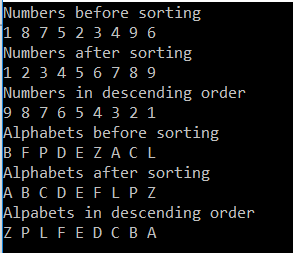# How to Sort a List of Simple Type in C#

## How to Sort a List of Simple Type in C#

In this article, I am going to discuss How to Sort a List of Simple Types in C# with Examples. Please read our previous article where we discussed the Range Methods of Generic List in C# class with examples.

##### How to Sort a List of Simple Types in C#?

In C#, sorting a list of simple types like int, double, char, string, etc. is straightforward. Here, we just need to call the Sort() method which is provided by the Generic List class on the list instance, and then the data will be automatically sorted in ascending order. For example, if we have a list of integers as shown below.
List<int> numbersList = new List<int>{ 1, 8, 7, 5, 2, 3, 4, 9, 6 };
Then we just need to invoke the Sort() method on numbers list collection as shown below
numbersList.Sort();
If you want the data to be retrieved in descending order, then use the Reverse() method on the list instance as shown below.
numbersList.Reverse();

However, when we do the same thing on a complex type like Employee, Product, Customer, Department, etc. we get a runtime exception as “invalid operation exception – Failed to compare 2 elements in the array”. This is because at runtime the .NET Framework does not identify how to sort the complex types. So, if we want to sort a complex type then we need to tell the way we want the data to be sorted in the list, and to do this we need to implement the IComparable interface. We will discuss this in the next article.

##### How the sort functionality is working for simple data types like int, double, string, char, etc. in C#?

This is working because these types (int, double, string, decimal, char, etc.) are already implementing the IComparable interface. If you go to the definition of any built-in types, then you will see that the class is implemented the IComparable interface. Let’s see an example to understand this concept.

```namespace ListCollectionSortReverseMethodDemo
{
public class Program
{
public static void Main()
{
List<int> numbersList = new List<int> { 1, 8, 7, 5, 2, 3, 4, 9, 6 };
Console.WriteLine("Numbers before sorting");
foreach (int i in numbersList)
{
Console.WriteLine(i);
}

// The Sort() of List Collection class
// will sort the data in ascending order
numbersList.Sort();
Console.WriteLine("Numbers after sorting");
foreach (int i in numbersList)
{
Console.WriteLine(i);
}

// If you want to  to retrieve data in descending order then use the
//Reverse() method of the List collection class
numbersList.Reverse();
Console.WriteLine("Numbers in descending order");
foreach (int i in numbersList)
{
Console.WriteLine(i);
}

//Another example of sorting alphabets
List<string> alphabets = new List<string>() { "B", "F", "P", "D", "E", "Z", "A", "C", "L" };
Console.WriteLine("Alphabets before sorting");
foreach (string alphabet in alphabets)
{
Console.WriteLine(alphabet);
}

alphabets.Sort();
Console.WriteLine("Alphabets after sorting");
foreach (string alphabet in alphabets)
{
Console.WriteLine(alphabet);
}

alphabets.Reverse();
Console.WriteLine("Alpabets in descending order");
foreach (string alphabet in alphabets)
{
Console.WriteLine(alphabet);
}